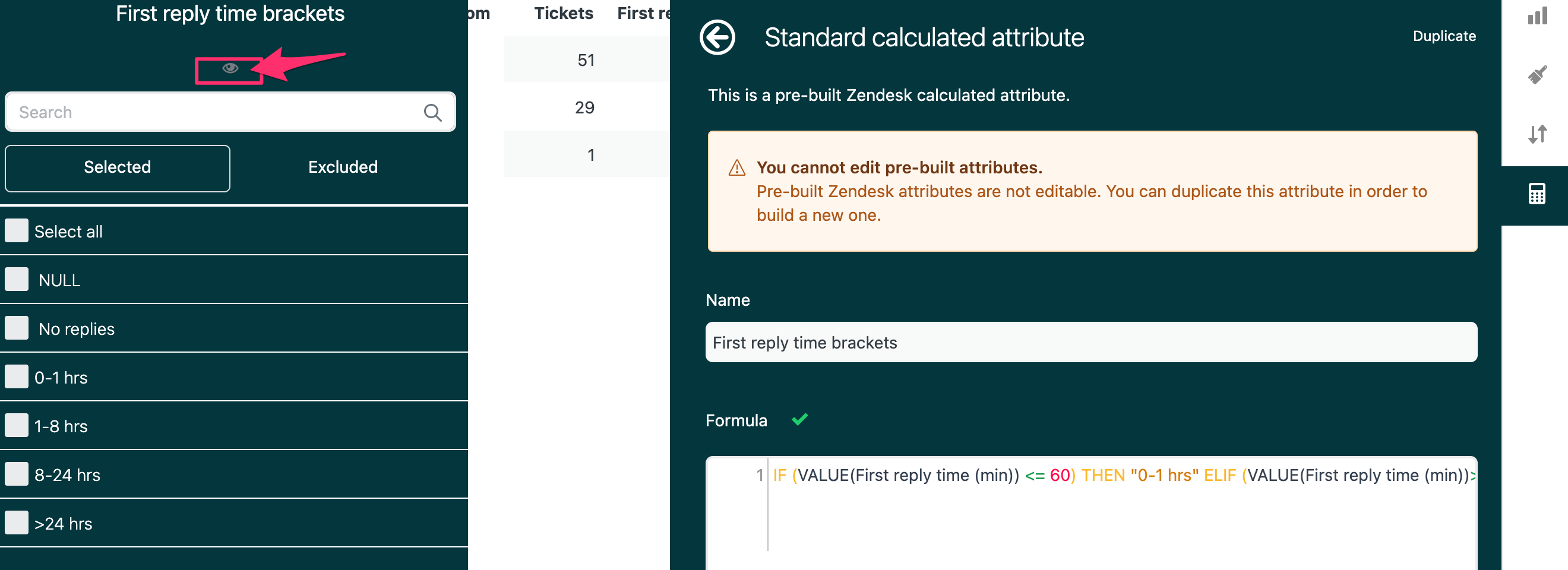# 2 コメント

Zendesk Customer Care

Hello Kate,

You can view the formula used for the default "First reply time brackets" if you click o the eye icon of the attribute.

From there, you can copy the formula, create a new custom attribute & edit it depending on how you want to break down the hours:In the break down of hours that you'd like to achieve, the formula should be like this:

``````IF (VALUE(First reply time (min)) <= 60) THEN "0-1 hrs"
ELIF (VALUE(First reply time (min))>60 AND VALUE(First reply time (min)) <= 60*4) THEN "1-4 hrs"
ELIF (VALUE(First reply time (min))>60*4 AND VALUE(First reply time (min)) <= 60*8) THEN "4-8 hrs"
ELIF (VALUE(First reply time (min))>60*8 AND VALUE(First reply time (min)) <= 60*16) THEN "8-16 hrs"
ELIF (VALUE(First reply time (min))>60*16 AND VALUE(First reply time (min)) <= 60*24) THEN "8-24 hrs"
ELIF (VALUE(First reply time (min)) > 60*24) THEN ">24 hrs"
ELSE " No replies"
ENDIF``````

Thank you!

サインインしてコメントを残してください。